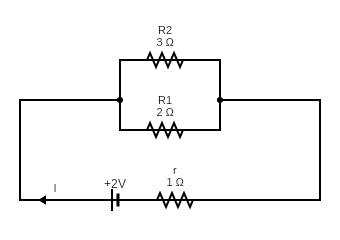Request a call back

A cell of EMF 2V and internal resistance 1ohm is connected with two wires of resistances 2ohm and 3ohm in parallel. Find the current in each wire.
Asked by www.utkarshdubey1408 | 20 Apr, 2022, 10:52: PMExpert Answer
Given that,
Internal resistance of cell, r = 1 Ohm
EMF of cell, V = 2 VNow,
The effective reisistance of wires connected in parallel combination will be given asHence the amount of current flowing through given combination will be
I = V(Rf + r)Now, the potential drop across parallel combination of wires will be
Vf = IRf =0.9 x 1.2 = 1.08 V
Thus,
Current through 3 Ohm resistance will be
I2 = Vf /R2 = 1.08 / 3 = 0.36 A
Similarly, Current through 2 Ohm resistance will be
I1 = Vf /R1 = 1.08 / 2 = 0.54 A
Answered by Jayesh Sah | 23 Apr, 2022, 08:11: PM
ICSE 10 - Physics
Asked by www.utkarshdubey1408 | 20 Apr, 2022, 10:52: PMANSWERED BY EXPERT
ICSE 10 - Physics
Calculate the current drawn from 2V battery in the diagram given below .Calculate VB - VAANSWERED BY EXPERT
ICSE 10 - Physics
Asked by saibaba1069 | 21 Jul, 2020, 07:23: PMANSWERED BY EXPERT
ICSE 10 - Physics
Asked by khushdab | 07 May, 2020, 12:18: PMANSWERED BY EXPERT
ICSE 10 - Physics
Asked by rohanraval2 | 09 Apr, 2020, 09:43: AMANSWERED BY EXPERT
ICSE 10 - Physics
Asked by najeebrafeena | 21 Sep, 2019, 01:32: PMANSWERED BY EXPERT
ICSE 10 - Physics
Asked by malapandey8278 | 08 Feb, 2019, 08:37: PMANSWERED BY EXPERT
ICSE 10 - Physics
Asked by Suryaraokondi1234 | 26 Jan, 2018, 04:48: AMANSWERED BY EXPERT